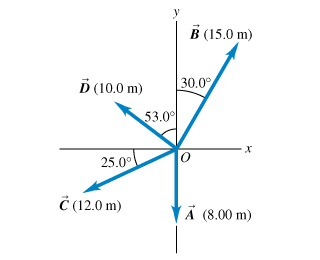Physics Practice Problems Vector Addition & Subtraction Practice Problems Solution: For the vectors A and B in the figure , use a scal...

⚠️Our tutors found the solution shown to be helpful for the problem you're searching for. We don't have the exact solution yet.

# Solution: For the vectors A and B in the figure , use a scale drawing to find the magnitude of the vector sum A+B .Find the magnitude of the vector difference A - B.Use your answers to find the magnitude of -A - B.Find the magnitude of B - A.Find the direction of the vector sum A+B .Find the direction of the vector difference A - B.Find the direction of -A - B.Find the direction of B - A.

###### Problem

For the vectors A and B in the figure, use a scale drawing to find the magnitude of the vector sum A+B .

Find the magnitude of the vector difference A - B.

Find the magnitude of B - A.

Find the direction of the vector sum A+B .

Find the direction of the vector difference A - B.

Find the direction of -A - B.

Find the direction of B - A.

#### Q. A spelunker is surveying a cave. She follows a passage that takes her a distance 181 m straight west, then a distance 211 m in a direction 45.0 eas...

Solved • Mon Oct 29 2018 18:49:54 GMT-0400 (EDT)

#### Q. A spelunker is surveying a cave. She follows a passage 160 m straight west, then 270 m in a direction 45 east of south, and then 280 m ...

Solved • Mon Oct 29 2018 18:49:52 GMT-0400 (EDT)